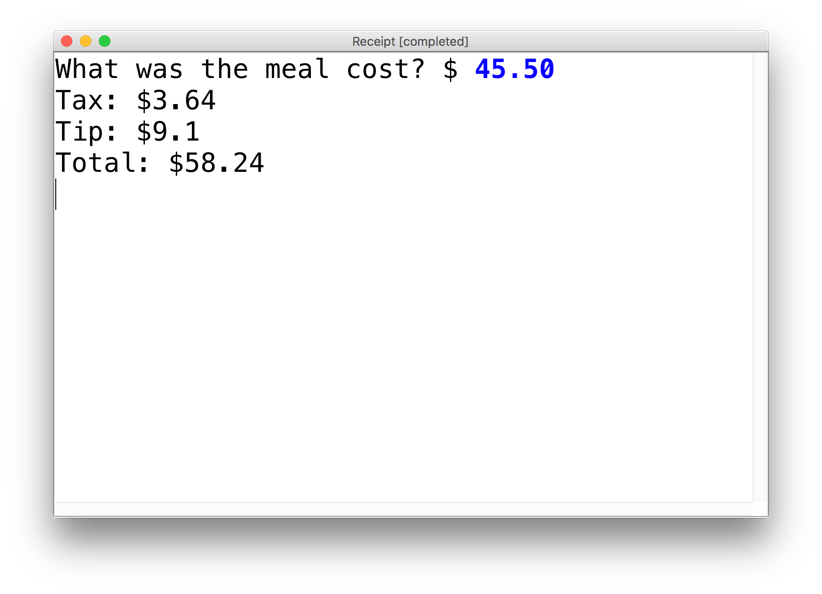Write a ConsoleProgram that calculates the tax, tip and total bill for us at a restaurant. The program should ask the user for the subtotal, and then calculate and print out the tax, tip and total.

Assume that tip is 20% and tax is 8% and that tip is on the original amount, not on the taxed amount.## Solution

```/**
* Program: Restaurant
* -------------
* This program helps restaurants calculate the bill
* taking into account tax and tip!
*/
public class Restaurant extends ConsoleProgram {

// This declares a constant... it can never change value.
private static final double TAX = 0.08; // Tax is 8%
private static final double TIP = 0.20; // Tip is 20%

public void run() {
// store the raw cost of the meal.
double cost = readDouble("What was the meal cost? \$");

double tax = cost * TAX;
double tip = cost * TIP;

double total = cost + tax + tip;

println("Tax: \$" + tax);
println("Tip: \$" + tip);
println("Total: \$" + total);
}
}```